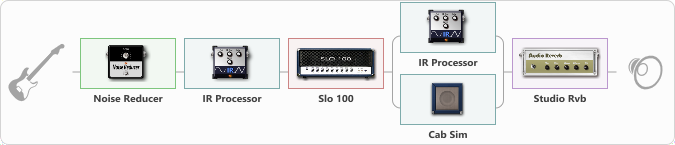# distor.solo

Discussion in 'ToneLib-GFX presets' started by carlinos3, Jan 9, 2022.

1. distor.solo

Preset name: Knife Fight

Effects chain:Effect: "Noise Reducer" (Dinámica / Filtro), active - "yes"
{
"Sens" = 74
"Mode" = Soft
}

Effect: "IR Processor" (Gabinetes), active - "yes"
{
"IR" = Forbidden Forbidden Evil
"Low Cut (Hz)" = 0
"Hi Cut (kHz)" = 20.0
"Mix" = 100
"Level (dB)" = 20
}

{
"Gain" = 39
"Bass" = 50
"Middle" = 100
"Treble" = 24
"Presence" = 42
"Master" = 78
"Level (dB)" = 2
}

Effect: "Splitter" (Dinámica / Filtro)
{
"A-Bypass" = Off
"A-Pan" = 0
"A-Level" = 55
"B-Bypass" = Off
"B-Pan" = 0
"B-Level" = 55

'A' branch:
{

Effect: "IR Processor" (Gabinetes), active - "yes"
{
"IR" = charv.1_dc
"Low Cut (Hz)" = 0
"Hi Cut (kHz)" = 20.0
"Mix" = 100
"Level (dB)" = 0
}
}
'B' branch:
{

Effect: "Cab Sim" (Gabinetes), active - "yes"
{
"Model" = 4x12" Greenback
"Level (dB)" = 0
}
}
}

Effect: "Studio Rvb" (Reverberación), active - "yes"
{
"Time" = 7.4
"PreDelay" = 32
"LoDamp" = 0
"HiDamp" = 12
"Mix" = 44
}

Note: You will need to download and install the ToneLib-GFX software to use the preset.

#### Attached Files:

• ###### distor.tlgfx
File size:
62.2 KB
Views:
2,208
JeremiahJohnson and yiğit like this.